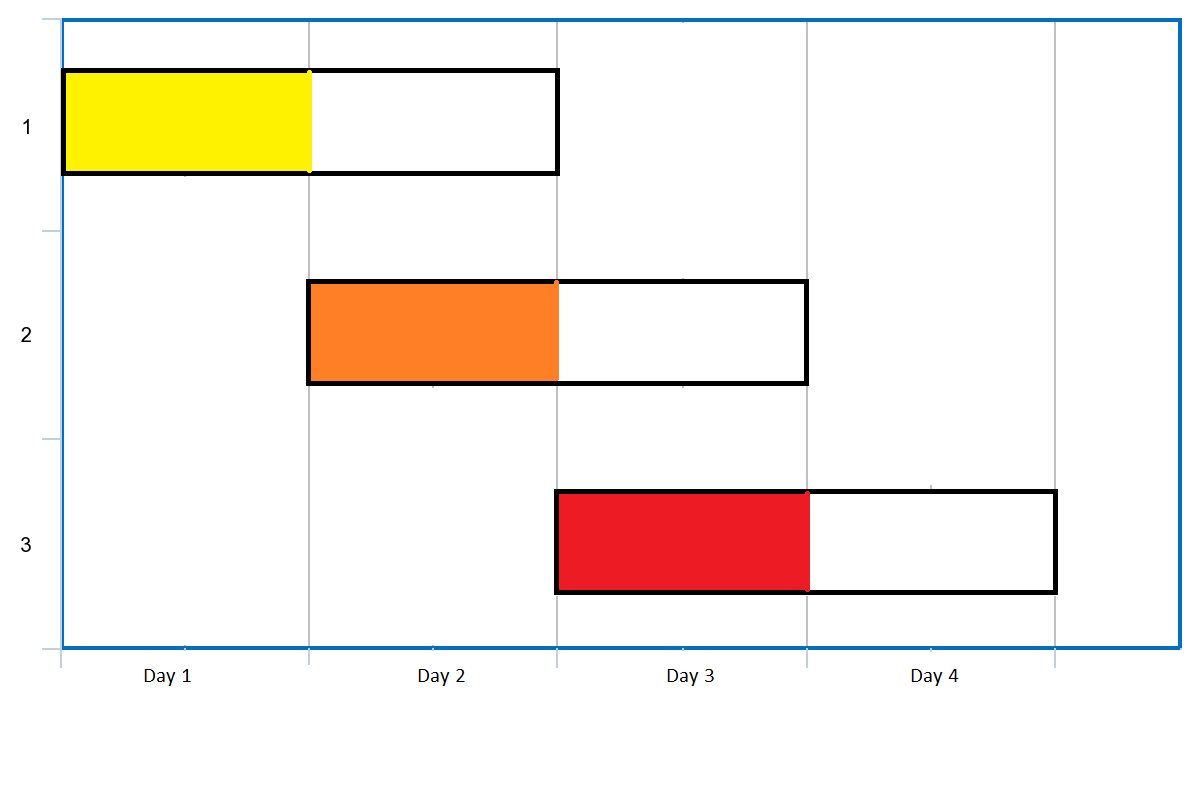# 5340. 统计有序矩阵中的负数

## 题目描述

输入：grid = [[4,3,2,-1],[3,2,1,-1],[1,1,-1,-2],[-1,-1,-2,-3]]



输入：grid = [[3,2],[1,0]]



输入：grid = [[1,-1],[-1,-1]]



输入：grid = [[-1]]



## 代码

class Solution {
public:
int countNegatives(vector<vector<int>>& grid) {
int m = grid.size();
int n = grid.size();
int cnt = 0;
for(int i = 0; i < m; ++i){
for(int j = 0; j < n; ++j){
if(grid[i][j] < 0){//统计负数的个数
++cnt;
}
}
}
return cnt;
}
};


# 5341. 最后 K 个数的乘积

## 题目描述

1. add(int num)

• 将数字 num 添加到当前数字列表的最后面。

2. getProduct(int k)

• 返回当前数字列表中，最后 k 个数字的乘积。
• 你可以假设当前列表中始终 至少 包含 k 个数字。

输入：
[[],,,,,,,,,,]

[null,null,null,null,null,null,20,40,0,null,32]

ProductOfNumbers productOfNumbers = new ProductOfNumbers();
productOfNumbers.getProduct(2); // 返回 20 。最后 2 个数字的乘积是 5 * 4 = 20
productOfNumbers.getProduct(3); // 返回 40 。最后 3 个数字的乘积是 2 * 5 * 4 = 40
productOfNumbers.getProduct(4); // 返回  0 。最后 4 个数字的乘积是 0 * 2 * 5 * 4 = 0
productOfNumbers.getProduct(2); // 返回 32 。最后 2 个数字的乘积是 4 * 8 = 32


## 代码1

class ProductOfNumbers {
public:
vector<int>vec;
ProductOfNumbers() {

}

vec.push_back(num);
}

int getProduct(int k) {
int len = vec.size(), res = 1;
for(int i = len - 1; i >= len - k; --i){
res = res * vec[i];
}
return res;
}
};

/**
* Your ProductOfNumbers object will be instantiated and called as such:
* ProductOfNumbers* obj = new ProductOfNumbers();
* int param_2 = obj->getProduct(k);
*/


0 <= num <= 100

## 代码2

class ProductOfNumbers {
public:
vector<int>vec;
ProductOfNumbers() {
vec.clear();
vec.push_back(1);
}

if(num == 0){//遇到0，清空前缀积数组
vec.clear();
vec.push_back(1);
}
else{
vec.push_back(vec.back() * num);
}
}
int getProduct(int k) {
if(vec.size() - 1 < k){//数组长度不足K表示，最后K个数中存在0
return 0;
}
else{
return vec[vec.size() - 1] / vec[vec.size() - 1 - k];
}
}
};

/**
* Your ProductOfNumbers object will be instantiated and called as such:
* ProductOfNumbers* obj = new ProductOfNumbers();
* int param_2 = obj->getProduct(k);
*/


# 1353. 最多可以参加的会议数目

## 题目描述输入：events = [[1,2],[2,3],[3,4]]



输入：events= [[1,2],[2,3],[3,4],[1,2]]



输入：events = [[1,4],[4,4],[2,2],[3,4],[1,1]]



输入：events = [[1,100000]]



输入：events = [[1,1],[1,2],[1,3],[1,4],[1,5],[1,6],[1,7]]



## 代码

class Solution {
public:
int maxEvents(vector<vector<int>>& events) {
priority_queue<int, vector<int>, greater<int>>que;
int cnt = 0, idx = 1, i = 0;
//先排序
sort(events.begin(), events.end());
while(i < events.size() || que.size() > 0){
while(i < events.size() && events[i] == idx){//开始时间为idx的，均添加到优先队列
que.push(events[i]);
++i;
}
while(que.size() > 0 && que.top() < idx){//去除安排冲突的
que.pop();
}
if(que.size() > 0){//优先安排结束时间早的
++cnt;
que.pop();
}
++idx;
}
return cnt;
}
};


# 5343. 多次求和构造目标数组

## 题目描述

• 令 x 为你数组里所有元素的和
• 选择满足 0  的任意下标 i ，并让 A 数组里下标为 i 处的值为 x 。
• 你可以重复该过程任意次

输入：target = [9,3,5]

[1, 1, 1], 和为 3 ，选择下标 1
[1, 3, 1], 和为 5， 选择下标 2
[1, 3, 5], 和为 9， 选择下标 0
[9, 3, 5] 完成


输入：target = [1,1,1,2]



输入：target = [8,5]



## 代码

class Solution {
public:
bool isPossible(vector<int>& target) {
long long int sum = 0;
int rep_num = 0;
bool flag = true;
priority_queue<int>que;
for(int i = 0; i < target.size(); ++i){
sum += target[i];
que.push(target[i]);
}
while(que.top() != 1){
sum = sum - que.top();//除去最大数字其它数字之和
if(sum == 1){
flag = true;
break;
}
/*
这里用除法而不用减法是为了处理像[100000, 1]这样的例子超时的问题
用除法，直接使当前的最大数字小于其它数字之和，避免多轮最大数字都是同一个。
*/
if(que.top() / sum == 0 || (que.top() % sum == 0)){//出现负数和0
flag = false;
break;
}
else{
que.push(que.top() % sum);
sum = sum + que.top() % sum;//上一个数组的所有元素之和
que.pop();
}
}
if(flag){
return true;
}
else{
return false;
}
}
};